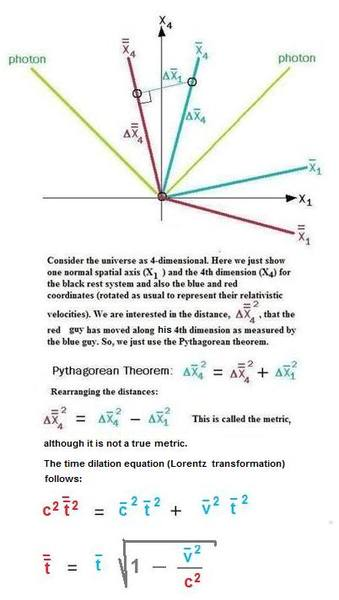# Lorentz transformation and Pythagoras' theorem

shounakbhatta
Hello,

I was just going through some writings on the web reg.Lorentz transformation.

Even considering the derivative factor,d, is anyway Lorentz transformation is some way, linked with Pythagoras' theorem?

Correct me if I am wrong?

-- Shounak

Staff Emeritus
Gold Member
The time dilation formula can be derived from an argument that uses the pythagorean theorem. See e.g. this page. Start reading at "The animation below..." Watch the animation (press the > button), and look at the triangle that appears at the end.

Last edited:
Homework Helper
One thing that is comonly done is to use the parameter $\tau= ict$ instead of time, t, so that the relativisitic "distance" $ds^2= dx^2+ dy^2+ dz^2- c^2dt$ becomes $ds^2= dx^2+ dy^2+ dz^2+ d\tau^2$, which, for simplicity, I will reduce to one space variable: $ds^2= dx^2+ d\tau^2$. That way the "hyperbolic" formula reduces to the Pythagorean theorem.

shounakbhatta
One thing I would like to make clear:

(a) Pythagoras' theorem:h^2=b^2+p^2 started with Euclidean plane

(b) Coming to 3 dimension, Minkowski re-wrote the formula as

h^2=b^2+p^2+x^2-(ct^2), where c is the speed of light and t the time co-ordinate

(c) In general relativity, or say, if we want to re-formulate Pythagoras' theorem on a sphere or curved surface, the length of the arm of the triangle can BE CONSIDERED flat in the infinitesimal calculus, hence we differentiate as:

dh^2=db^2+dp^2+dx^2-c^2dt^2

Please correct me if I am wrong.

Thanks,

-- Shounak

Staff Emeritus
Gold Member
It doesn't have anything to do with curvature or general relativity, and that last formula isn't just a matter of differentiation. It's pretty hard to explain what that formula is about in non-mathematical terms.

bobc2
Hello,

I was just going through some writings on the web reg.Lorentz transformation.

Even considering the derivative factor,d, is anyway Lorentz transformation is some way, linked with Pythagoras' theorem?

Correct me if I am wrong?

-- Shounak

Here is a derivation of time dilation based on the Pythagorean Theorem. It begins with the proposition that all observers measure the same value for the speed of light, regardless of their relative speeds. For this to be true, one picture of such a universe logically includes four dimensions; different observers moving at different constant relativistic speeds relative to each other would be associated with 4-dimensional worldlines slanted with respect to an arbitrarily selected rest frame. An observer's worldline is colinear with his X4 axis along the 4th dimension ("time axis"). Further, the X1 axis is slanted as well, such that the 45-degree photon world line always bisects the angle between X1 and X4 (this assures the same measurement of "c" in all inertial frames)--that's just the way the universe is constructed in four dimensions. In the sketch below, the X1 axes represent the cross-section of the 4-dimensional universe (X2 and X3 are suppressed for ease of viewing) experienced by an observer at some instant of time (some point along the X4 dimension). This sets up the geometry in which you apply the Pythagorean Theorem. In this sketch a blue guy and red guy move in opposite directions at the same relativistic speed relative to the black rest frame. We regard any observer to be moving along his own X4 axis at the speed of light: X4 = ct, or t = X4/cLast edited: